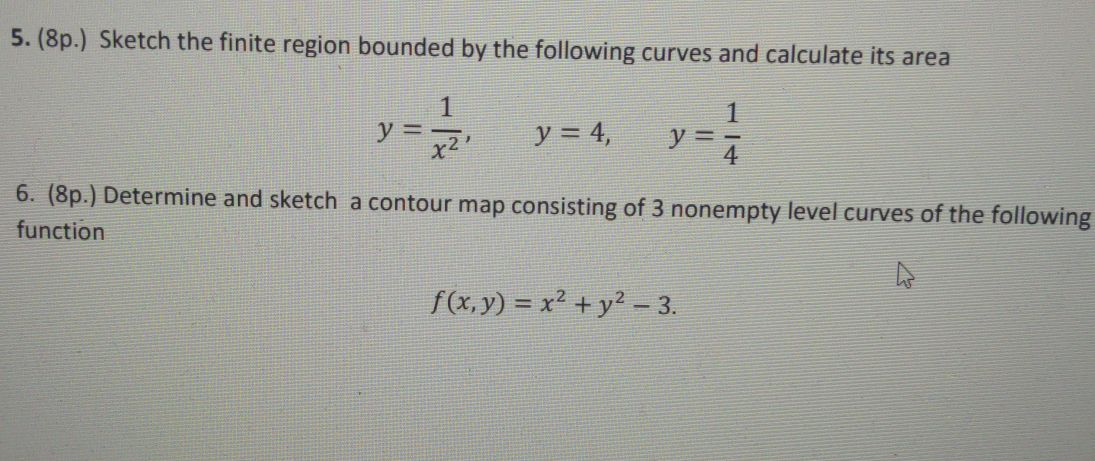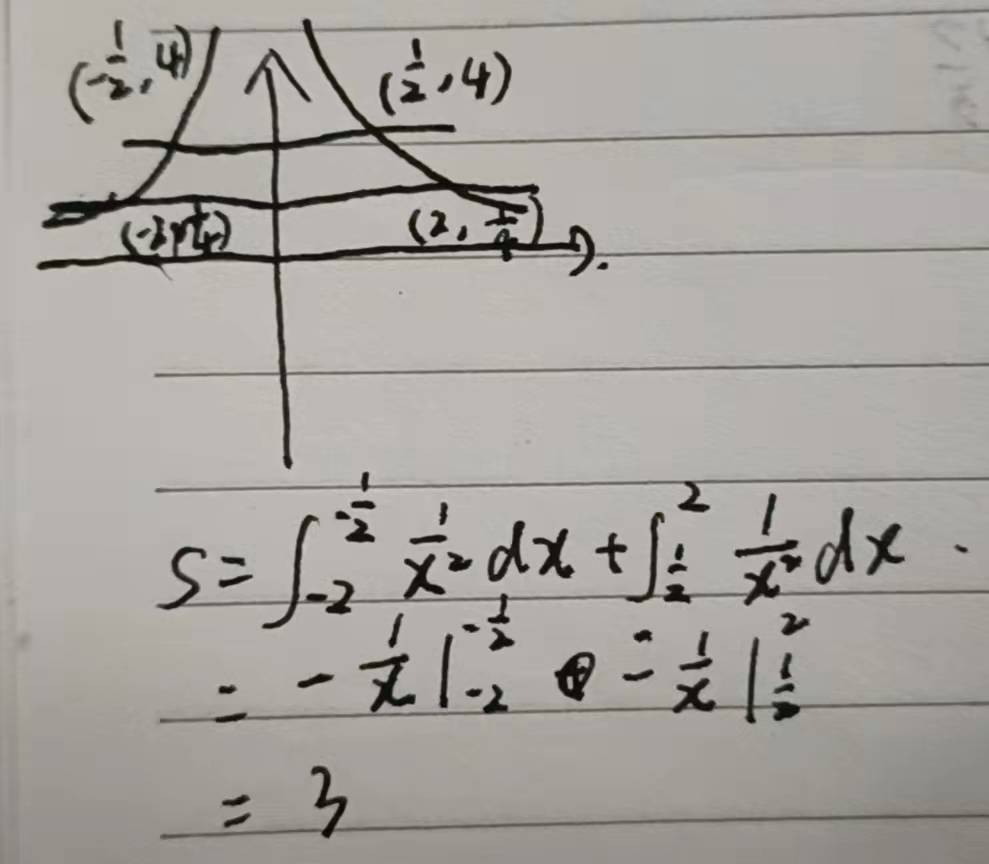### ¿Todavía tienes preguntas de matemáticas?

Pregunte a nuestros tutores expertos
Algebra
Pregunta5. (8p.) Sketch the finite region bounded by the following curves and calculate its area

$$y = \frac { 1 } { x ^ { 2 } } , y = 4 , y = \frac { 1 } { 4 }$$

6. (8p.) Determine and sketch a contour map consisting of $$3$$ nonempty level curves of the following function

$$f ( x , y ) = x ^ { 2 } + y ^ { 2 } - 3$$